본문바로가기

## 검색결과

검색결과 (전체 1건)

### 검색결과제한

열기
• 소장도서관
• 원문
• 발행년도
-
• 언어
• 원문이용안내
•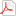모든 이용자
•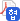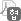협정기관 이용자
•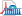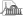국회도서관 방문 이용자
• 원문아이콘이 없는 자료는 국회도서관에 방문하여 책자로만 이용이 가능합니다.

논문명/저자명
Electronic Properties of TiO₂(110) and Ru/Pt(111) Surfaces : A Molecular Orbital Study of H₂O Adsorption/ 이춘기
발행사항
부산: 경성대학교 대학원, 2002.8
청구기호
TD 541.3 ㅇ915e
형태사항
107 p. ; 26 cm
자료실
전자자료
제어번호
KDMT1200255295
주기사항
학위논문(박사) -- 경성대학교 대학원, 화학, 2002.8
원문
미리보기

목차보기더보기

CONTENTS

1. Introduction 10

References 19

2. Theoretical background 22

2.1. Extended Huckel theory 22

2.1.1. The approach 22

2.1.2. Mulliken population analysis 25

2.1.3. The charge matrix 31

2.2. Counterintuitive orbital mixing 33

2.3. The extension of extended Huckel theory to extended systems 40

2.3.1. Constructing the hamiltonian and overlap matrix 42

2.3.2. k-points and the Brillouin Zone 47

2.3.3. The fermi level average energy and total density of states 53

2.3.4. The charge matrix, AO occupations and projected densities of states 54

2.3.5. Crystal orbital overlap populations 56

References 60

3. Electronic properties of Ru/Pt(111) alloy surface: a theoretical study of H2O adsorption 63

3.1. Introduction 63

3.2. Theoretical model 64

3.3. Electronic properties of pure Pt and Pt-Ru alloy surfaces 68

3.5. Discussion and conclusions 83

References 87

4. Molecular orbital analysis of water activation on TiO2(110) surface 90

4.1. Introduction 90

4.2. Rutile TiO2(110) surface and H2O molecule 93

4.3. Results and discussion 95

4.3.1. Electronic properties of the bare TiO2(110) surface 95

4.3.2. Chemisorption of H2O 99

4.3.3. Dissociation of H2O on TiO2(110) 103

4.4. Conclusion 114

References 115

감사의 말씀 119

Table 2.1 Comparison of Mulliken overlap populations with experimental bond dissociation enthalpies (BDEs). The default eHT parameters were used for all calculations Table 2.2 Calculated values for the AB model system. E: MO energy, C: Coefficient matrix 5

Table 2.2 Calculated values for the AB model system. E: MO energy, C: Coefficient matrix elements, CM: Charge matrix elements, red AB P : AB reduced overlap population between atoms A and B, Pop: Net AO occupations. The columns correspond to calculations with (no COM)# 35

Table 3.1 Parameters used for the calculations 65

Table 3.2 Bonding characteristics for H2O adsorption on Pt(111) and Pt-Ru alloy clusters as modeled in Fig. 3.1 71

Table 4.1 Atomic parameters used in the calculations 81

Table 4.2 Electron densities and overlap populations for the bare TiO2(110) surface and the TiO2(110)/H2O surface 98

Table 4.3 Electron densities and overlap populations for the bare TiO2(110)surface and the TiO2(110)/H2O(t) surface 105

Figure 2.1 Calculated Mulliken overlap populations plotted as a function of experimental BDE for a number of diatomic molecules. 30

Figure 2.2 The effects of counterintuitive orbital mixing (COM) on the shapes of the molecular orbitals of a two-orbital system. The relative positions of the energy levels are only approximate. 30

Figure 2.3 The lowest energy orbital of the three-orbital AAB molecule. (a) and (b) show the orbital from non-weighted and weighted H calculations respectively. 38

Figure 2.4 A sketch of the first Brillouin Zone (BZ) of a square lattice (the region bounded by the dashed box) and its irreducible wedge (the shaded region). The high-symmetry points of the BZ are labeled: Γ =(0,0) ; X= (½,0) or (0, ½), M= (½, ½) P1-4, 39

Figure 2.5 A sketches of: (a) a square lattice with a square basis; (b) a square lattice with a rectangular basis; (c) a rectangular lattice with a square basis. The real space lattice (top), reciprocal lattice (bottom), first BZ (bottom, dashed box) and irreducible w2 50

Figure 3.1 Two-layer cluster (a) and slab (b) models of the Pt-Ru alloy used for the calculations. The dark circles indicate the substitutional Ru atoms in the Pt(111) surface 65

Figure 3.2 DOS projected on the Pt d (dotted line) and s (dashed line) orbitals in pure Pt (a) and DOS projected on the Pt d (dotted line) and the Ru d (dashed line) orbitals in the alloy (b). The solid line represents the total DOS. The dashed vertical line refers toC 67

Figure 3.3 COOP of the Pt-Pt bond in pure Pt (a) and COOP of the Ru- Pt bond in the alloy (b). The dashed vertical line refers to the Fermi level. 70

Figure 3.4 Schematic illustration showing the stabilization of a ligand (L) lone-pair orbital by a metal (M) surface orbital. Note the destabilizing component indicated by the heavy upward pointing arrow. If the metal surface orbital is empty, there will be no destabiJ 74

Figure 3.5 Orbital interactions between H2O and a surface metal atom in the on-top site. 75

Figure 3.6 H2O adsorption on Pt(111): DOS projected on the dz 2 (a) and dyz (b) (dotted line) of a Pt atom of the adsorption site and on the pz(O) (a) and py(O) (b) (dashed line) of H2O, and COOP of the Pt-O bond (c). The vertical bars display the position of 3a1 and M 77

Figure 3.7 H2O adsorption on Pt in the alloy: DOS projected on the dz 2 (a) and dyz (b) (dotted line) of a Pt atom of the adsorption site and on the pz(O) (a) and py(O) (b) (dashed line) of H2O, and COOP of the Pt-O bond (c). The vertical bars display the position of O 79

Figure 3.8 H2O adsorption on Ru in the alloy: DOS projected on the dz 2 (a) and dyz (b) (dotted line) of a Ru atom of the adsorption site and on the pz(O) (a) and py(O) (b) (dashed line) of H2O, and COOP of the Ru-O bond (c). The vertical bars display the position ofP 80

Figure 4.1. The (Ti15O36)12- cluster used to study the H2O adsorption on TiO2(110). Ob represents the bridging oxygens, Op the in-plane oxygens, Ti(5) the fivefold-coordinated titaniums, and Ti(6) the sixfoldcoordinated titaniums. 94

Figure 4.2 Density of states of TiO2(110). The dotted and dashed lines represent the PDOS on the Ti and Ob atoms, respectively. The vertical dashed line refers to the Fermi level. 94

Figure 4.3 (a) DOS of H2O at Ti(5). (b) DOS of the isolated H2O monolayer. The vertical dashed line refers to the Fermi level. 100

Figure 4.4 The overlap populations of O.H (○) and Ob.H (●) as a function of tilt angle of the H2O.Ti(5) bond from the surface normal for H2O chemisorption at the Ti(5) site on TiO2(110). 102

Figure 4.5 PDOS, and O.H (solid line) and Ob.H (dotted line) COOP curves for the H2O adsorbed in a tilted conformation ( θ=35°) The dashed line refers to the Fermi level. 107

Figure 4.6 Evolution of 2b1 orbital at two selected O-H distances of one OH bond, (a) 0.957 and (b) 1.157 Å, for the H2O molecule adsorbed in a tilted conformation ( θ=25°). The dashed line refers to the Fermi level. 110

## 권호기사보기

권호기사 목록 테이블로 기사명, 저자명, 페이지, 원문, 기사목차 순으로 되어있습니다.
기사명 저자명 페이지 원문 기사목차
연속간행물 팝업 열기 연속간행물 팝업 열기